## How to Convert Polar to Cartesian | Coordinate Units

The image below represents polar to cartesian.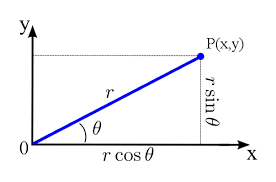To convert polar to cartesian, three essential parameters are needed and these parameters are the Value of r and Value of θ.

The formula for converting polar to cartesian (x, y):

x = rcosθ

y = rsinθ

Let’s solve an example;
Find the conversion of polar to cartesian when the value of r is 4 and value of θ is 8.

This implies that;

Value of r = 4
Value of θ = 8

Finding x:

x = 4 x cos(8°)
x = 4 x 0.99
x = 3.96

Therefore, is 3.96.

Finding y:

y = 4 x sin(8°)
y = 4 x 0.139
y = 0.55

Therefore, y is 0.55.

Therefore, the Cartesian coordinates of the polar coordinates (4∠8°) is (3.96, 0.55).

## How to Convert Cartesian to Polar | Coordinate Units

The image below represents cartesian to polar.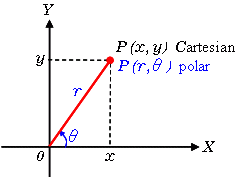To convert cartesian to polar, three essential parameters are needed and these parameters are the Value of x and Value of y.

The formula for converting cartesian to polar (r∠θ):

r = √(x² + y²)

θ = tan-1(y / x)

Let’s solve an example;
Find the conversion of cartesian to polar when the value of x is 5 and value of y is 10.

This implies that;

Value of x = 5
Value of y = 10

Finding r:

r = √(5² + 10²)
r = √(25 + 100)
r = √(125)
r = 11.18

Therefore, is 11.18

Finding θ:

θ = tan-1(10 / 5)
θ = tan-1(2)
θ = 63.43°

Therefore, θ is 63.43°.

Therefore, the polar coordinates of the Cartesian coordinates (5, 10) is 11.18∠(63.43°).

## How to Convert Spherical to Cartesian | Coordinate Units

The image below represents spherical to cartesian.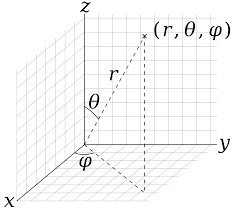To convert spherical to cartesian, three essential parameters are needed and these parameters are the Value of r, the Value of θ, and the Value of φ.

The formula for converting spherical to cartesian (x, y, z):

x = rsinθcosφ

y = rsinθsinφ

z = rcosθ

Let’s solve an example;
Find the conversion of spherical to cartesian when the value of r is 2, the value of θ is 8 and the value of φ is 4.

This implies that;

Value of r = 2
Value of θ = 8
Value of φ = 4

Finding x:

x = 2 x sin(8°) x cos(4°)
x = 2 x 0.139 x 0.997
x = 0.277

Therefore, is 0.277.

Finding y:

y = 2 x sin(8°) x sin(4°)
y = 2 x 0.139 x 0.069
y = 0.019

Therefore, is 0.0019.

Finding z:

z = 2 x cos(8°)
z = 2 x 0.9902680687415704
z = 1.98

Therefore, z is 1.98.

Therefore, the Cartesian coordinates of the spherical coordinates (2, 8°, 4°) is (0.27, 0.019, 1.98).

## How to Convert Spherical to Cylindrical | Coordinate Units

The image below represents spherical to cylindrical.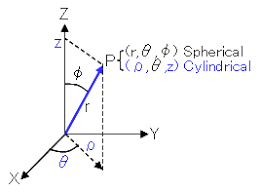To convert spherical to cylindrical, three essential parameters are needed and these parameters are the Value of r, the Value of θ, and the Value of φ.

The formula for converting spherical to cylindrical (ρ, φ, z):

φ = rsinθ

φ = φ

z = rcosθ

Let’s solve an example;
Find the conversion of spherical to cylindrical when the value of r is 3, the value of θ is 9 and the value of φ is 2.

This implies that;

Value of r = 3
Value of θ = 9
Value of φ = 2

Finding ρ:

ρ = 3 x sin(9°)
ρ = 3 x 0.156
ρ = 0.469

Therefore, ρ is 0.469.

Finding φ:

φ = 2°

Therefore, φ is 2.

Finding z:

z = 3 x cos(9°)
z = 3 x 0.987
z = 2.963

Therefore, z is 2.963.

Therefore, the cylindrical coordinates of the spherical coordinates (3, 9°, 2°) is (0.469, 2°, 2.963).

## How to Convert Cylindrical to Spherical | Coordinate Units

The image below represents cylindrical to spherical.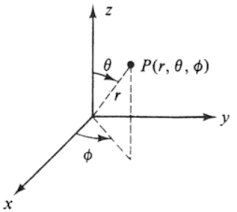To convert cylindrical to spherical, three essential parameters are needed and these parameters are the Value of ρ, the Value of φ, and the Value of z.

The formula for converting cylindrical to spherical (r, θ, φ):

r = √(φ² + z²)

θ = tan-1(ρ / z)

φ = φ

Let’s solve an example;
Find the conversion of cylindrical to cartesian when the value of ρ is 2, the value of φ is 3 and the value of z is 5.

This implies that;

Value of ρ = 2
Value of φ = 3
Value of z = 5

Finding r:

r = √(2² + 5²)
r = √(4 + 25)
r = √(29)
r = 5.38

Therefore, is 5.38

Finding θ:

θ = tan-1(2 / 5)
θ = tan-1(0.4)
θ = 21.80°

Therefore, θ is 21.80°.

Finding φ:

φ = 3°

Therefore, the spherical coordinates of the cylindrical coordinates (2, 3°, 5) is (5.38, 21.80°, 3°).

## How to Convert Cylindrical to Cartesian | Coordinate Units

The image below represents cylindrical to cartesian.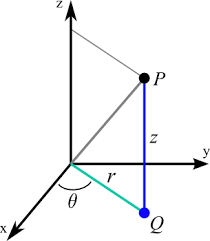To convert cylindrical to cartesian, three essential parameters are needed and these parameters are the Value of ρ, the Value of φ, and the Value of z.

The formula for converting cylindrical to cartesian (x, y, z):

x = ρcosφ

y = ρsinφ

z = z

Let’s solve an example;
Find the conversion of cylindrical to cartesian when the value of ρ is 3, the value of φ is 6 and the value of z is 7.

This implies that;

Value of ρ = 3
Value of φ = 6
Value of z = 7

Finding x:

x = 3 x cos(6°)
x = 3 x 0.99
x = 2.98

Therefore, is 2.98

Finding y:

y = 3 x sin(6°)
y = 3 x 0.1045
y = 0.313

Therefore, is 0.313

Finding z:

z = 7

Therefore, the Cartesian coordinates of the cylindrical coordinates (3, 6°, 7) is (2.98, 0.313, 7).

## How to Convert Cartesian to Spherical | Coordinate Units

The image below represents cartesian to spherical.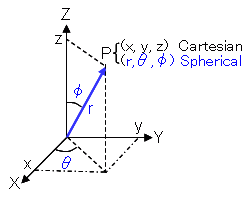To convert cartesian to spherical, three essential parameters are needed and these parameters are the Value of x, the Value of y, and the Value of z.

The formula for converting cartesian to spherical (r, θ, φ):

r = √(x² + y² + z²)

φ = tan-1(y / x)

θ = tan-1((√(x² + y²) / z)

Let’s solve an example;
Find the conversion of cartesian to spherical when the value of x is 4, the value of y is 3 and the value of z is 6.

This implies that;

Value of x = 4
Value of y = 3
Value of z = 6

Finding r:

r = √(4² + 3² + 6²)
r = √(16 + 9 + 36)
r = √(61)
r = 7.81

Therefore, r is 7.81

Finding θ:

θ = tan-1((√(4² + 3²)) / 6)
θ = tan-1((√(16 + 9)) / 6)
θ = tan-1((√(25)) / 6)
θ = tan-1((5) / 6)
θ = tan-1(0.8333333333333334)
θ = 39.80°

Therefore, θ is 39.80°.

Finding φ:

φ = tan-1(3 / 4)
φ = tan-1(0.75)
φ = 36.86°

Therefore, φ is 36.86°.

Therefore, the spherical coordinates of the Cartesian coordinates (4, 3, 6) is (7.81, 39.80°, 36.86°).

## How to Convert Cartesian to Cylindrical | Coordinate Units

The image below represents cartesian to cylindrical.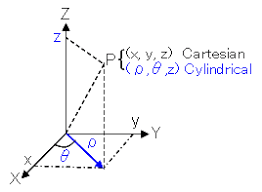To convert cartesian to cylindrical, three essential parameters are needed and these parameters are the Value of x, the Value of y, and the Value of z.

The formula for converting cartesian to cylindrical (ρ, φ, z):

ρ = √(x² + y²)

φ = tan-1(y / x)

z = z

Let’s solve an example;
Find the conversion of cartesian to cylindrical when the value of x is 2, the value of y is 4 and the value of z is 8.

This implies that;

Value of x = 2
Value of y = 4
Value of z = 8

Finding ρ:

ρ = √(2² + 4²)
ρ = √(4 + 16)
ρ = √(20)

Therefore, ρ is 4.47.

Finding φ:

φ = tan-1(4 / 2)
φ = tan-1(2)
φ = 63.43494882292201°

Therefore, φ is 63.43°.

Finding z:

z = 8

Therefore, the cylindrical coordinates of the Cartesian coordinates (2, 4, 8) is (4.47, 63.43°, 8).

## How to Convert Square Miles to Acres | Area Units

The image below represents square miles to acres.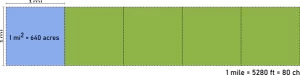To convert square miles to acres, one essential parameter is needed and this parameter is Unit Value.

The formula for converting square miles to acres:

1 Square Mile = 640 Acres

Let’s solve an example;
Find the conversion of square miles to acres when the unit value is 4.

1 Square Mile = 640 Acres

Then,

4 Square Miles = (4 x 640) Acres
4 Square Miles = 2560 Acres

Therefore, the acre(s) is 2560.

## How to Convert Square Miles to Square Yards | Area Units

The image below represents square miles to square yards.To convert square miles to square yards, one essential parameter is needed and this parameter is Unit Value.

The formula for converting square miles to square yards:

1 Square Mile = 3097600 Square Yards

Let’s solve an example;
Find the conversion of square miles to square yards when the unit value is 30.

1 Square Mile = 3097600 Square Yards

Then,

30 Square Miles = (30 x 3097600) Square Yards
30 Square Miles = 92928000 Square Yards

Therefore, the square yard(s) is 92928000.